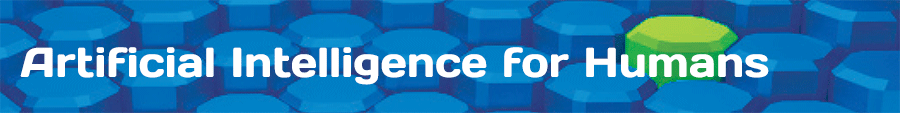AIFH Volume 3, Chapter 6: Neural Network Classification

Network Type:
Patterns:

This example makes use of a feedforward neural network to demonstrate classification. To make use of this application draw several colored dots onto the drawing area. Make sure you have at least two colors, or there will be nothing to classify. Once you have drawn something click begin, and the neural network will begin to train. You will see how other regions near the data points you provided are classified.

The above neural network has two input neurons and three output neurons. The hidden layer structure is defined by the drop list. For example, if you choose 2:10:10:3, you will have a network that looks like the following image. This network has two hidden layers, with 10 neurons each.The input neurons represent the x and y coordinates of a dot. To draw the above image the program loops over a grid of x and y coordinates. The neural network is queried for each of the grid components. The cell in the upper left is [0,0], the cell in the lower right is [1,1]. Data to a neural network with sigmoid activation functions should usually receive input in the range between 0 and 1, so this range works fine. The center would be [0.5,0.5].

The output from the neural network represents the RGB color that that grid square should have. The value of [0,0,0] would represent black, and the value [1,1,1] would represent white.

As you draw on the drawing region you are providing training data. The input neurons will represent the x and y coordinate that you placed the data at. The expected, or ideal, output will represent the color that you chose for that location.

Lets look at a simple example. If you draw only two data points, then the area will be divided in half. Here you can see a red and blue data point provided.For the application to get the error level low it only needs to make sure that the blue data point is in a blue region, and the red data point is in a red region. All other points are "guessed" based on the other points. With such a very small amount of data, it is difficult for the neural network to really guess where the border between the two zones actually is.

If you provide more training data you will get a more complex shape. If you chose to create a two-color random image, will you will be given data points similar to the following.Here the neural network creates a much more complex pattern to try to fit around all of the data points.

You might also choose to create a complex multi-color pattern. Here random colors were generated for the points. The neural network will even blend colors to try to compromise and lower the error as much as it can.It is even possible to learn complex inter-winding shapes such as the following.